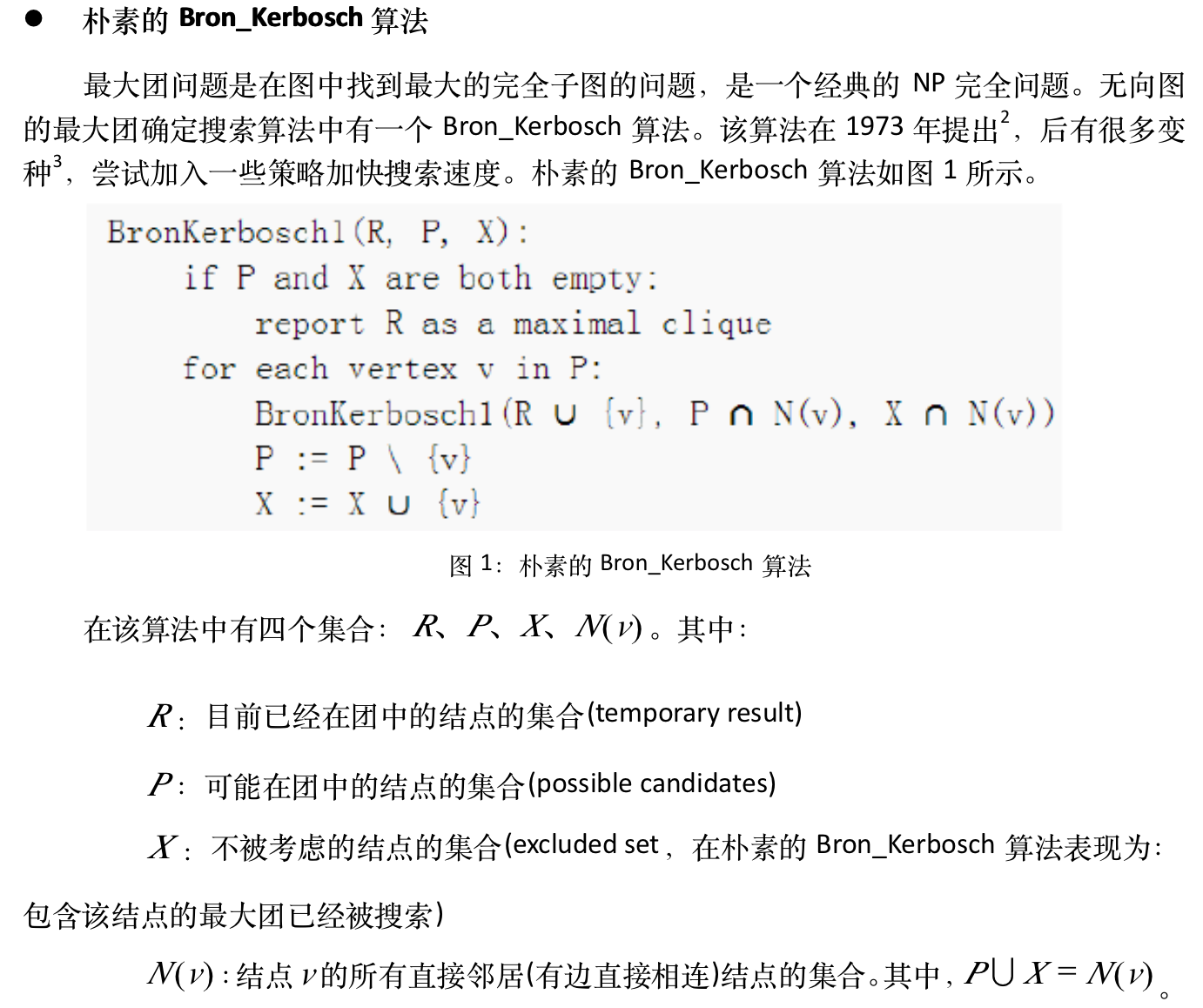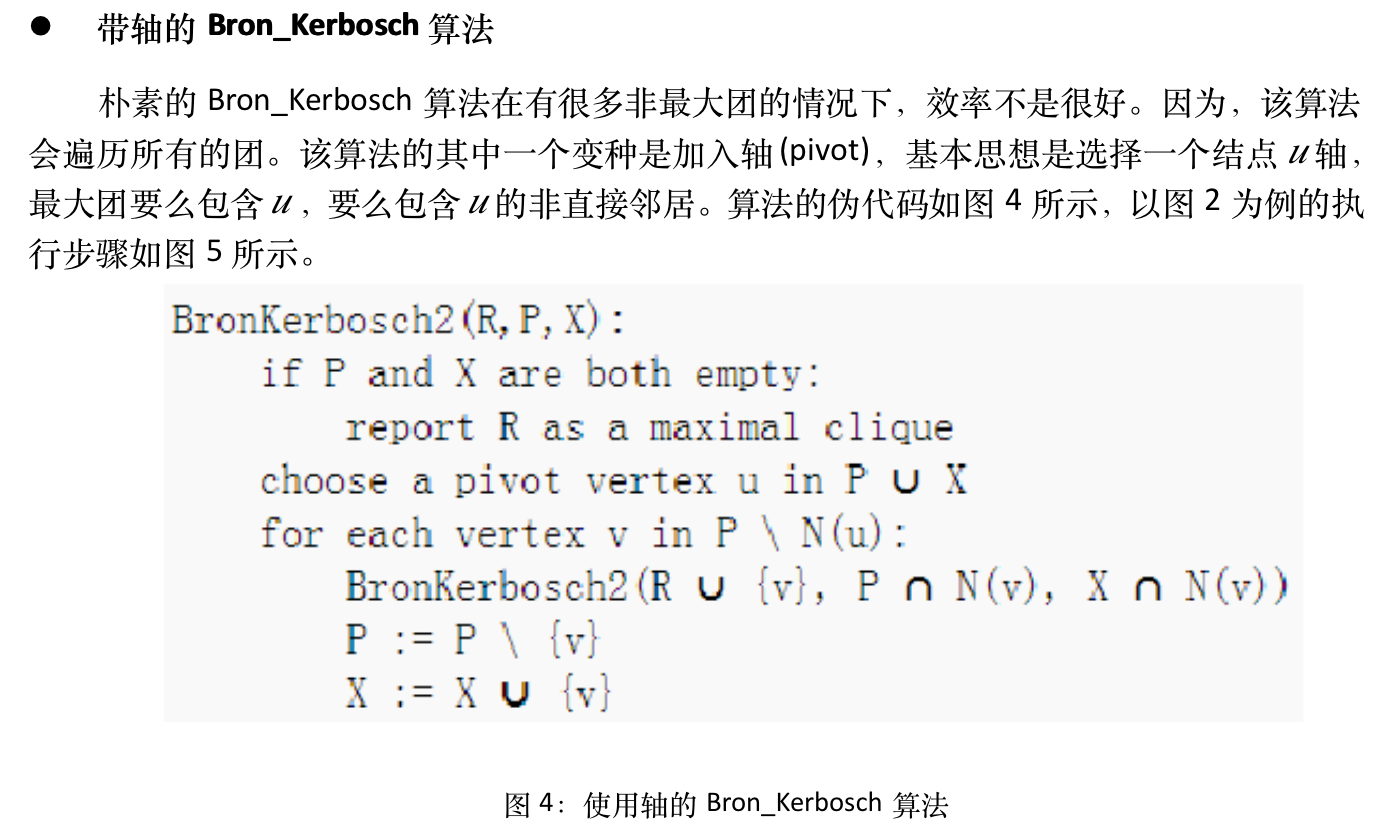# Codeforces 839E Mother of Dragons（最大团）

E. Mother of Dragons
time limit per test
2 seconds
memory limit per test
256 megabytes
input
standard input
output
standard output

There are n castles in the Lannister's Kingdom and some walls connect two castles, no two castles are connected by more than one wall, no wall connects a castle to itself.

Sir Jaime Lannister has discovered that Daenerys Targaryen is going to attack his kingdom soon. Therefore he wants to defend his kingdom. He has k liters of a strange liquid. He wants to distribute that liquid among the castles, so each castle may contain some liquid (possibly zero or non-integer number of liters). After that the stability of a wall is defined as follows: if the wall connects two castles a and b, and they contain x and y liters of that liquid, respectively, then the strength of that wall is x·y.

Your task is to print the maximum possible sum of stabilities of the walls that Sir Jaime Lannister can achieve.

Input

The first line of the input contains two integers n and k (1 ≤ n ≤ 401 ≤ k ≤ 1000).

Then n lines follows. The i-th of these lines contains n integers ai, 1, ai, 2, ..., ai, n (). If castles i and j are connected by a wall, then ai, j = 1. Otherwise it is equal to 0.

It is guaranteed that ai, j = aj, i and ai, i = 0 for all 1 ≤ i, j ≤ n.

Output

Print the maximum possible sum of stabilities of the walls that Sir Jaime Lannister can achieve.

Your answer will be considered correct if its absolute or relative error does not exceed 10 - 6.

Namely: let's assume that your answer is a, and the answer of the jury is b. The checker program will consider your answer correct, if.

Examples
input
3 1
0 1 0
1 0 0
0 0 0

output
0.250000000000

input
4 4
0 1 0 1
1 0 1 0
0 1 0 1
1 0 1 0

output
4.000000000000

Note

In the first sample, we can assign 0.5, 0.5, 0 liters of liquid to castles 1, 2, 3, respectively, to get the maximum sum (0.25).

In the second sample, we can assign 1.0, 1.0, 1.0, 1.0 liters of liquid to castles 1, 2, 3, 4, respectively, to get the maximum sum (4.0)

有一个无向图，和一个数字K，要求把K把K分配到一些结点上（可以分配小数），使得每个边两边的点的值乘积之和最大。

可以发现，把K均等分给最大团上的点时答案最优。对于最大团的求解，一个比较快的方法是Bron_Kerbosch算法。

关于Bron_Kerbosch算法的介绍就直接引用别人的了。AC代码：

#include <algorithm>
#include <cstdio>
using namespace std;
#define ULL unsigned long long

const int MAXN=45;//最大结点数，根据题意修改
int V;//顶点数
int max_clique;//最大团的大小
ULL G[MAXN];//邻接矩阵的状压表示

inline int ctz(ULL s)//返回末尾0的个数，由于__bilitin_ctzll输入0时未定义，这里手动定义
{
return s?__builtin_ctzll(s):64;
}

void dfs(ULL R,ULL P,ULL X)//已经在团中的点状压，可能在团中的点状压，不考虑的点状压
{
if(!P && !X)//当前团是极大团
{
max_clique=max(max_clique,__builtin_popcountll(R));//R中1的就是极大团中点，如果要输出构成最大团的点，这里更新时保存R中的点即可
return ;
}
if(!P)//当前团是以求得的极大团的子图
return;
int pivot = ctz(P|X);//选择一个轴点
ULL PP = P&~G[pivot];//代选择点中不与轴点相邻的点
for(int u=ctz(PP);u<V;u+=ctz(PP>>(u+1))+1)//枚举PP中的点
{
dfs(R|((ULL)1<<u),P&G[u],X&G[u]);
P ^= (ULL)1<<u;//把u移到不考虑的点中
X |= (ULL)1<<u;
}
}

int BronKerbosch()
{
max_clique=0;
dfs(0,((ULL)1<<V)-1,0);//初始团中没有点，所有点都可能在团中，没有点不考虑
return max_clique;
}

int main()
{
int K;
scanf("%d%d", &V, &K);
for(int i=0;i<V;++i)
for(int j=0;j<V;++j)
{
int tmp;
scanf("%d", &tmp);
if(tmp)
G[i]|=(ULL)1<<j;
}
int i = BronKerbosch();
printf("%.12lf\n", 1.0*K*K*(i-1)/i/2.0);

return 0;
}